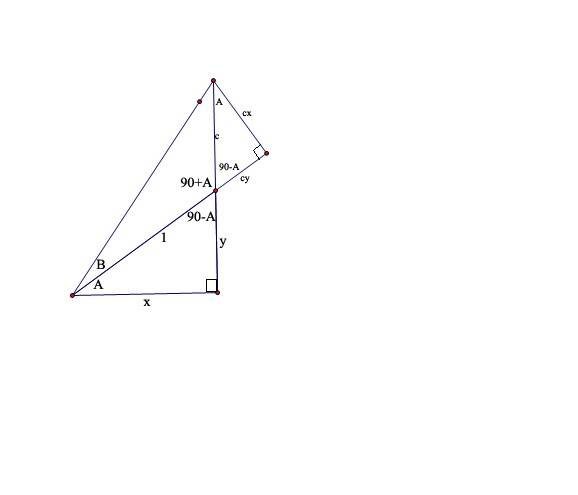Need proof

Need proof!!!

Will any one provide me the proof for the expansions of
1) sin(A+B)
2)sin(A-B)
3)cos(A+B)
4)cos(A-B)

Thanks in advance....CompuChip
Homework Helper

2) and 4) follow from 1) and 3) respectively, when you use that sin(-x) = -sin(x) and cos(-x) = cos(x) in addition.

1) and 3) can be proven by geometric arguments (try Google ;) )

The classic development of these formulae that I've seen follows these lines:

Begin with cos(a - b). Draw a unit circle centered at O = (0, 0) with a point at A = (1, 0) and three others labeled B = (x1, y1), C = (x2, y2), and D = (x3, y3) on the circumference situated at angles b, a - b, and a radians respectively. Since the angles COA and DOB are both (a - b) then the distances CA and DB must be the same. Calculating these distances leads to the derivation of cos(a - b), which is x2.

cos(a + b) follows from cos(a - (-b)).

sin(a - b) follows from cos((π/2 - a) + b) via co-fuction identity sin(u) = cos(π/2 - u).

sin(a + b) follows from sin(a - (-b)).

There are other derivations of cos(a - b) than the one I gave above.

If this isn't clear enough, I will try to hammer out a daigram with proof.

--Elucidus

Write them down as e-functions:

$$\sin(\alpha + \beta) = \frac{i}{2}\left(e^{-i(\alpha + \beta)}-e^{i(\alpha + \beta)}\right)=\frac{i}{2}\left(e^{-i\alpha}e^{-i\beta}-e^{i\alpha}e^{i\beta}\right)$$

Now remember:

$$e^{i\varphi}=\cos \varphi + i\sin \varphi$$

Substitute it, and the result falls on you feet. The rest is proved mutatis mutandis.

The classic development of these formulae that I've seen follows these lines:

Begin with cos(a - b). Draw a unit circle centered at O = (0, 0) with a point at A = (1, 0) and three others labeled B = (x1, y1), C = (x2, y2), and D = (x3, y3) on the circumference situated at angles b, a - b, and a radians respectively. Since the angles COA and DOB are both (a - b) then the distances CA and DB must be the same. Calculating these distances leads to the derivation of cos(a - b), which is x2.

cos(a + b) follows from cos(a - (-b)).

sin(a - b) follows from cos((π/2 - a) + b) via co-fuction identity sin(u) = cos(π/2 - u).

sin(a + b) follows from sin(a - (-b)).

There are other derivations of cos(a - b) than the one I gave above.

If this isn't clear enough, I will try to hammer out a daigram with proof.

--Elucidus

I think the diagram will be better.....So please prove this diagramatically...

Thanks in advancejgens
Gold Member

Well, since he/she has already outlined an approach, why don't you try drawing a diagram and completing the proof?

Here is an illustration that may get you going in the right direction. You will need the following:

$$\text{distance between two points }d=\sqrt{(x_i-x_j)^2+(y_i-y_j)^2}$$

and since each of the points is on the perimeter of the unit circle

$$x_i^2+y_i^2=1$$.

I hope this helps

--Elucidus

EDIT: The point A in the illustration should be (1, 0), not (0, 1). Oops.

Attachments

•Cosine (A - B).gif
3.2 KB · Views: 473
Last edited:

First consider the attached diagram, convince yourself of its validity. Please note that since the small right triangle at the top has angle A, then the length of its sides must be a scalar multiple of the right triangle with hypotenuse 1.

Clearly, by the Pythagorean theorem the hypotenuse of the right triangle with angle $$A+B$$ is equal to $$$\sqrt{(1+cy)^2 +c^2x^2}$$$
Now, considering the large right triangle we see that
$$\sin (A+B) = \frac{y+c}{\sqrt{(1+cy)^2 +c^2x^2}}$$
But we also see that
$$$sin A = y, sin B = \frac{cx}{\sqrt{(1+cy)^2 +c^2x^2}}$ $cos A = x, cos B = \frac{1+cy}{\sqrt{(1+cy)^2 +c^2x^2}}$$$
and so
$$$sin A cos B + sin B cos A = \frac{y+cy^2}{\sqrt{(1+cy)^2 +c^2x^2}} + \frac{cx^2}{\sqrt{(1+cy)^2 +c^2x^2}} = \frac{y + c(x^2 + y^2)}{\sqrt{(1+cy)^2 +c^2x^2}} = \frac{y+c}{\sqrt{(1+cy)^2 +c^2x^2}} = sin (A+B)$$$
since $$x^2 + y^2 = 1$$
And the rest can be found in similar ways. The main problem with this proof is that it only works for angles whose sum is less than 90 degrees. The key to generalizing it would be to say that the diagram below holds for any sum of angles, because the reference angle of the sum is less than 90 degrees, and so such a triangle can always be constructed. I have not yet entirely convinced myself of it yet, but I thought it was a neat proof anyway!CompuChip